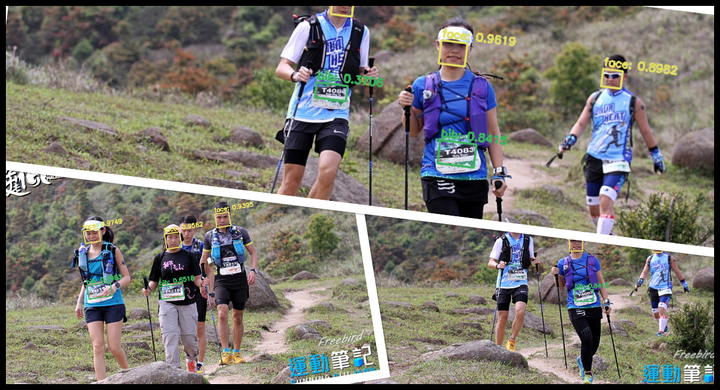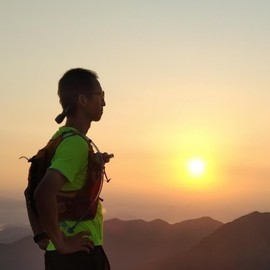# Bib Racer 03 - Face and Bib Detection with YOLO networkI just made a very simple face and bib detection program following the post by Adrian Rosebrock, with the weights trained with the downloaded trail running images using method described in the previous post. The speed is not very fast, which take more than 1 second for an image. Certainly, it is Google Colab free tier, so there are lots of variables that we cannot control and even do not know. I’ll try to come back to this issue later since speed is not a big concern in the moment.

Before we can start training YOLO model for bib and face detection, we need a training set for the task and I have prepared 501 training images with the help of labelImg. LabelImg has a nice UI and a simple workflow. It supports to output the annotations to PASCAL VOC and YOLO format. The annotation process is tedious but necessary. Here is part of the training set to give you some idea.Some training images that will be tagged for faces and bibs in YOLO format

So here it is, first we load set all the necessary parameters and class labels.

from google.colab.patches import cv2_imshow
import numpy as np
import cv2

# default parameters
confidence_threshold = 0.5
nms_threshold = 0.25

meta_dir = data_path+"yolo_cfg/"
labels = open(meta_dir+"obj.names").read().strip().split("\n")

Then we are going to load the YOLO weights and configuration file by OpenCV dnn module, and find out the output layers to get the detection results.

# load YOLO weights and configuration file
cfg = meta_dir+"yolo-obj.cfg"
weight = data_path+"weights_backup/yolo-obj_4000.weights"
# load YOLO detector trained on custom dataset

# determine the output layer names
l_names = net.getLayerNames()
ol_names = [l_names[i-1] for i in net.getUnconnectedOutLayers()]

Next, we load the image and feed it to YOLO model for detection.

# load the image
image_path = data_path+"images/valid_tiny_50files/1024_621C72DC-115C-8FFF-DF8B-66F8C302C598.JPG"
#import time
if image is not None:
(H,W) = image.shape[:2]
# construct a blob from the input image, pass to the YOLO detector and
# grab the bounding boxes and associated probabilities
blob = cv2.dnn.blobFromImage(image, 1/255.0, (416,416), swapRB=True, crop=False)
net.setInput(blob)
#start = time.time()
layer_outputs = net.forward(ol_names)
#end = time.time()
#print("Time: {:.6f}".format(end-start))
else:
print("No image is read.")

Once we have the results, we only pick detections with confidence higher than the predefined value and assign a box to each of them, and perform Non-maximum suppression on those boxes.

boxes = []
confidences = []
classIDs = []

# output of YOLO [0:4]: [center_x, center_y, box_w, box_h]
# output of YOLO : confidence
# output of YOLO [5:]: class scores
for output in layer_outputs:
for detection in output:
scores = detection[5:]
classID = np.argmax(scores)
confidence = scores[classID]

if confidence > confidence_threshold:
(center_x, center_y, width, height) = (detection[0:4] * ([W, H, W, H])).astype("int")
x = int(center_x - (width/2))
y = int(center_y - (height/2))
boxes.append([x, y, int(width), int(height)])
confidences.append(float(confidence))
classIDs.append(classID)

# perform Non-Maximum Suppression
idxs = cv2.dnn.NMSBoxes(boxes, confidences, confidence_threshold, nms_threshold)

And finally, we would like to display the image with the detected faces and bibs, as well as the corresponding confidences.

# fancy: initialize a list of colors to represent each possible class label
np.random.seed(42)
COLORS = np.random.randint(0, 255, size=(len(labels), 3),	dtype="uint8")

if len(idxs) > 0:
for i in idxs.flatten():
(x,y) = (boxes[i], boxes[i])
(w,h) = (boxes[i], boxes[i])
color = [int(c) for c in COLORS[classIDs[i]]]
cv2.rectangle(image, (x,y), (x+w, y+h), color, 2)
text = "{}: {:.4f}".format(labels[classIDs[i]], confidences[i])
cv2.putText(image, text, (x, y-5), cv2.FONT_HERSHEY_SIMPLEX, 0.5, color, 2)

cv2_imshow(image)

Here are the detection results of the same image with different confidence threshold:Confidence threshold = 0.5. Some faces and bibs are missed out.